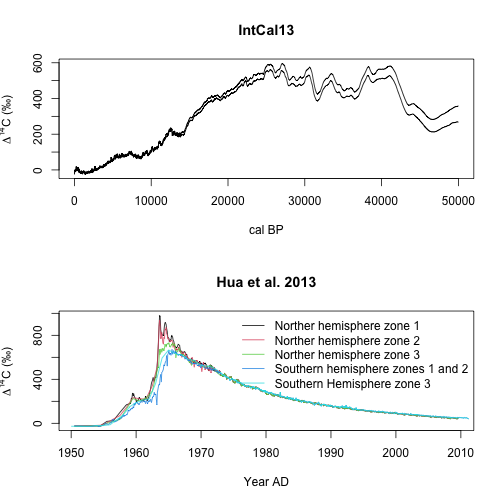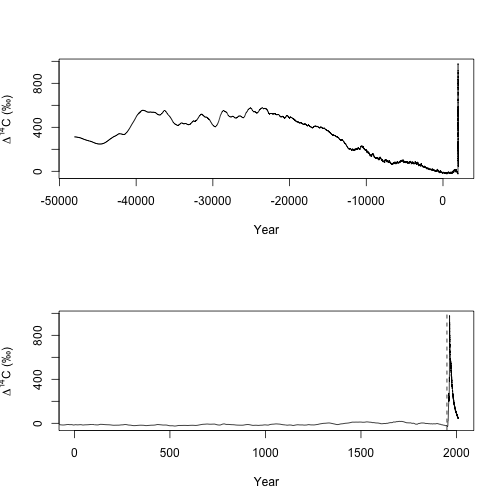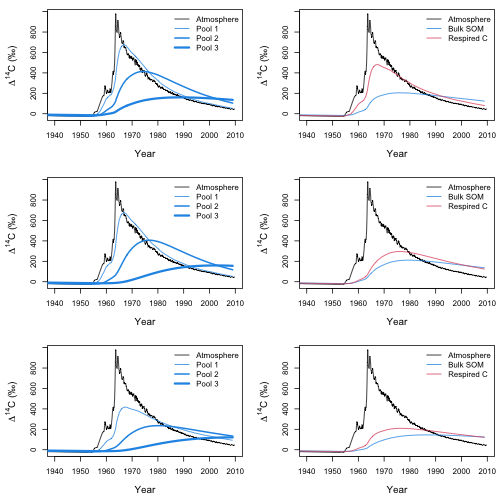In this workflow we will implement examples of multiple-pool radiocarbon models, in particular examples from Sierra et al. (2014) as well as procedures to manipulate atmospheric radiocarbon curves.

## General model structure

We will deal here with linear compartment models of the form

$\frac{\mathrm{d} \mathbf{C}(t)}{\mathrm{d}t} = \mathbf{I}(t) + \mathbf{A}(t) \mathbf{C}(t), \qquad \mathbf{C}(t=0) = \mathbf{C_0}$ that has a radiocarbon counterpart as $\frac{\mathrm{d}\mathbf{^{14}C}(t)}{\mathrm{d}t} = \mathbf{I}_{^{14}C}(t) + \mathbf{A}(t) \mathbf{^{14}C}(t) - \lambda \mathbf{^{14}C}(t)$ where $$\lambda$$ is the radioactive decay constant. This radiocarbon model can also be expressed in terms of the activity ratio of sample $$F$$, so

$\frac{\mathrm{d} (\mathbf{F}(t) \circ \mathbf{C}(t))}{\mathrm{d}t} =F_\mathrm{atm}(t) \mathbf{I}(t) + \mathbf{A}(t)\,(\mathbf{F}(t) \circ \mathbf{C}(t)) - \lambda\,(\mathbf{F}(t) \circ \mathbf{C}(t))$ where the symbol $$\circ$$ represents the entry-wise product among vectors, and $$F_\mathrm{atm}(t)$$ is the atmospheric radiocarbon over time. This means that for running radiocarbon models we need a dataset of atmospheric radiocarbon values.

There are two main internationally accepted radiocarbon datasets that shows the historical behavior of 14C in the atmosphere. For the pre-modern period before 1950, the IntCal13 dataset is the current standard (Reimer et al. 2013). This dataset is included by default in SoilR in the object IntCal13. For the post-bomb period, the dataset of Hua et al. (2013) is included in the SoilR object Hua2013.

We can see both datasets:

par(mfrow=c(2,1))
plot(IntCal13$CAL.BP,IntCal13$Delta.14C+IntCal13$Sigma,type="l", main="IntCal13", xlab="cal BP",ylab=expression(paste(Delta^14,"C (\u2030)"))) lines(IntCal13$CAL.BP,IntCal13$Delta.14C-IntCal13$Sigma)

plot(Hua2013$NHZone1$Year.AD, Hua2013$NHZone1$mean.Delta14C, main="Hua et al. 2013",
lines(Hua2013$NHZone2$Year.AD,Hua2013$NHZone2$mean.Delta14C,col=2)
lines(Hua2013$NHZone3$Year.AD,Hua2013$NHZone3$mean.Delta14C,col=3)
lines(Hua2013$SHZone12$Year.AD,Hua2013$SHZone12$mean.Delta14C,col=4)
lines(Hua2013$SHZone3$Year.AD,Hua2013$SHZone3$mean.Delta14C,col=5)
legend(
"topright",
c(
"Norther hemisphere zone 1",
"Norther hemisphere zone 2",
"Norther hemisphere zone 3",
"Southern hemisphere zones 1 and 2",
"Southern Hemisphere zone 3"
),
lty=1,
col=1:5,
bty="n"
)In many cases, it is useful to combine both atmospheric curves for long-term simulations that include both the pre- and post-bomb periods. SoilR includes the function bind.C14curves for this purpose.

For example, to bind the IntCal13 curve with the Hua2013 curve for the northern hemisphere zone 1:

ad=bind.C14curves(prebomb=IntCal13,postbomb=Hua2013\$NHZone1,time.scale="AD")

par(mfrow=c(2,1))
abline(v=1950,lty=2)par(mfrow=c(1,1))


Notice that due to the long-time scale it is sometimes useful to plot just a portion of the dataset such as in the second panel of the previous figure.

## Three-pool example

We will implement now three different versions of a three-pool model with different connections among the pools. For each model, we will calculate the radiocarbon content in the pools as well as the radiocarbon content in the bulk soil and the released C.

We will run simulations starting in the year 1901 until year 2009 and will have a total amount of litter inputs to the soil of 100 units of mass. Each pool will start with a C content of 100, 500, and 1000, mass units respectively, and will decompose at three different rates in units of yr$$^{-1}$$:

years=seq(1901,2009,by=0.5)
LitterInput=100
k=c(k1=1/2, k2=1/10, k3=1/50)
C0=c(100,500,1000)



A parallel model structure can then be implemented with the function ThreepParallelModel_14. We need to specify the initial radiocarbon content for each pool, and partitioning coefficients gam of the incoming litter:

Parallel=ThreepParallelModel14(
t=years,
ks=k,
C0=C0,
F0_Delta14C=c(0,0,0),
In=LitterInput,
gam1=0.6,
gam2=0.2,
inputFc=Fatm
)


A series model structure can be implemented with the function ThreepSeriesModel_14, assuming all litter enters the first pool and it's transferred to the second pool according to a coefficient a21, and from the second to the third pools according to a coefficient a32:

a21=0.9*k #90% from fast to intermediate pool
a32=0.4*k #40 from intermediate to slow pool

Series=ThreepSeriesModel14(
t=years,
ks=k,
C0=C0,
F0_Delta14C=c(0,0,0),
In=LitterInput,
a21=a21,
a32=a32,
inputFc=Fatm
)


A feedback model structure is implemented with the function ThreepFeedbackModel_14, with transfers coefficients from the second to first pool a12 and from the third to the second pool a23:

a12=0.4*k
a23=0.7*k

Feedback=ThreepFeedbackModel14(
t=years,
ks=k,
C0=C0,
F0_Delta14C=c(0,0,0),
In=LitterInput,
a21=a21,
a12=a12,
a32=a32,
a23=a23,
inputFc=Fatm
)


To calculate the average radiocarbon content for the bulk soil we will use the function getF14C, and for the average radiocarbon of the release flux the function getF14R. The radiocarbon content of each individual pool is calculated with the function getF14.

# Parallel model
P.R14m=getF14R(Parallel)
P.C14m=getF14C(Parallel)
P.C14t=getF14(Parallel)

# Series model
S.R14m=getF14R(Series)
S.C14m=getF14C(Series)
S.C14t=getF14(Series)

# Feedback model
F.R14m=getF14R(Feedback)
F.C14m=getF14C(Feedback)
F.C14t=getF14(Feedback)


Now we are ready to plot the results

par(mfrow=c(3,2), mar=c(5,5,1,1),cex.lab=1.25)
plot(Fatm,
type="l",
xlab="Year",
ylab=expression(paste(Delta^14,"C ","(\u2030)")),
xlim=c(1940,2010)
)
lines(years, P.C14t[,1], col=4)
lines(years, P.C14t[,2],col=4,lwd=2)
lines(years, P.C14t[,3],col=4,lwd=3)
legend(
"topright",
c("Atmosphere", "Pool 1", "Pool 2", "Pool 3"),
lty=rep(1,4),
col=c(1,4,4,4),
lwd=c(1,1,2,3),
bty="n"
)

plot(Fatm,type="l",xlab="Year",
ylab=expression(paste(Delta^14,"C ","(\u2030)")),xlim=c(1940,2010))
lines(years,P.C14m,col=4)
lines(years,P.R14m,col=2)
legend("topright",c("Atmosphere","Bulk SOM", "Respired C"),
lty=c(1,1,1), col=c(1,4,2),bty="n")

plot(Fatm,type="l",xlab="Year",
ylab=expression(paste(Delta^14,"C ","(\u2030)")),xlim=c(1940,2010))
lines(years, S.C14t[,1], col=4)
lines(years, S.C14t[,2],col=4,lwd=2)
lines(years, S.C14t[,3],col=4,lwd=3)
legend("topright",c("Atmosphere", "Pool 1", "Pool 2", "Pool 3"),
lty=rep(1,4),col=c(1,4,4,4),lwd=c(1,1,2,3),bty="n")

plot(Fatm,type="l",xlab="Year",
ylab=expression(paste(Delta^14,"C ","(\u2030)")),xlim=c(1940,2010))
lines(years,S.C14m,col=4)
lines(years,S.R14m,col=2)
legend("topright",c("Atmosphere","Bulk SOM", "Respired C"),
lty=c(1,1,1), col=c(1,4,2),bty="n")

plot(Fatm,type="l",xlab="Year",
ylab=expression(paste(Delta^14,"C ","(\u2030)")),xlim=c(1940,2010))
lines(years, F.C14t[,1], col=4)
lines(years, F.C14t[,2],col=4,lwd=2)
lines(years, F.C14t[,3],col=4,lwd=3)
legend("topright",c("Atmosphere", "Pool 1", "Pool 2", "Pool 3"),
lty=rep(1,4),col=c(1,4,4,4),lwd=c(1,1,2,3),bty="n")

plot(Fatm,type="l",xlab="Year",
ylab=expression(paste(Delta^14,"C ","(\u2030)")),xlim=c(1940,2010))
lines(years,F.C14m,col=4)
lines(years,F.R14m,col=2)
legend("topright",c("Atmosphere","Bulk SOM", "Respired C"),
lty=c(1,1,1), col=c(1,4,2),bty="n")Notice that these three different model structure lead to very different temporal behaviors of the radiocarbon content in the soil, in the release flux, and in each individual pool.